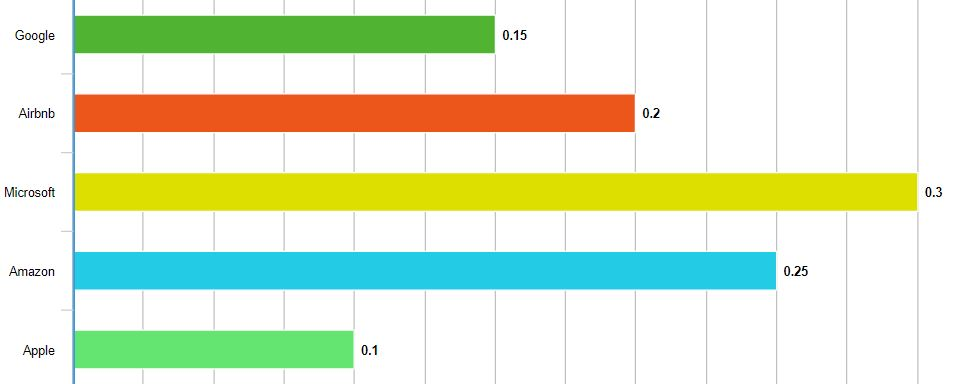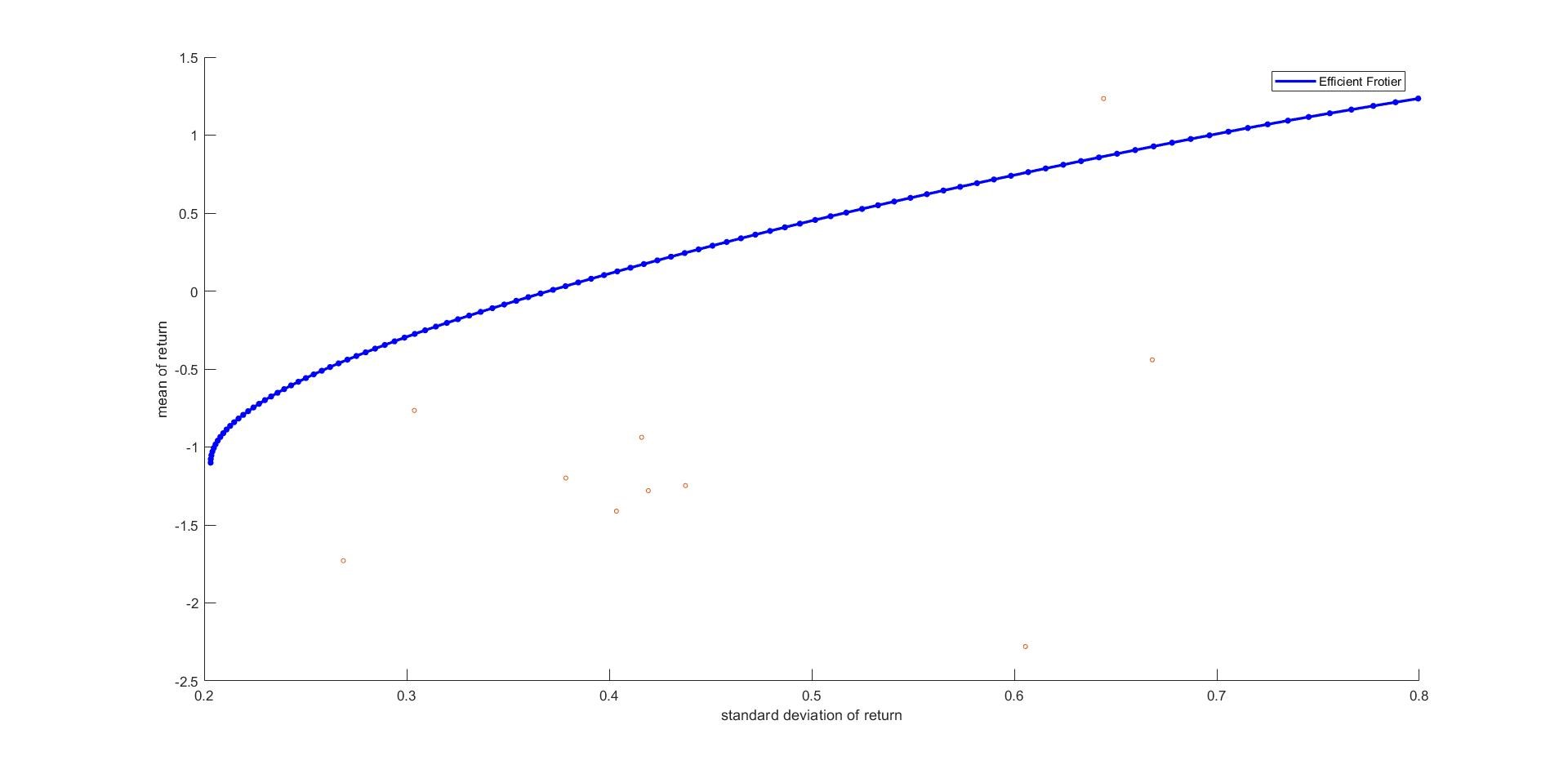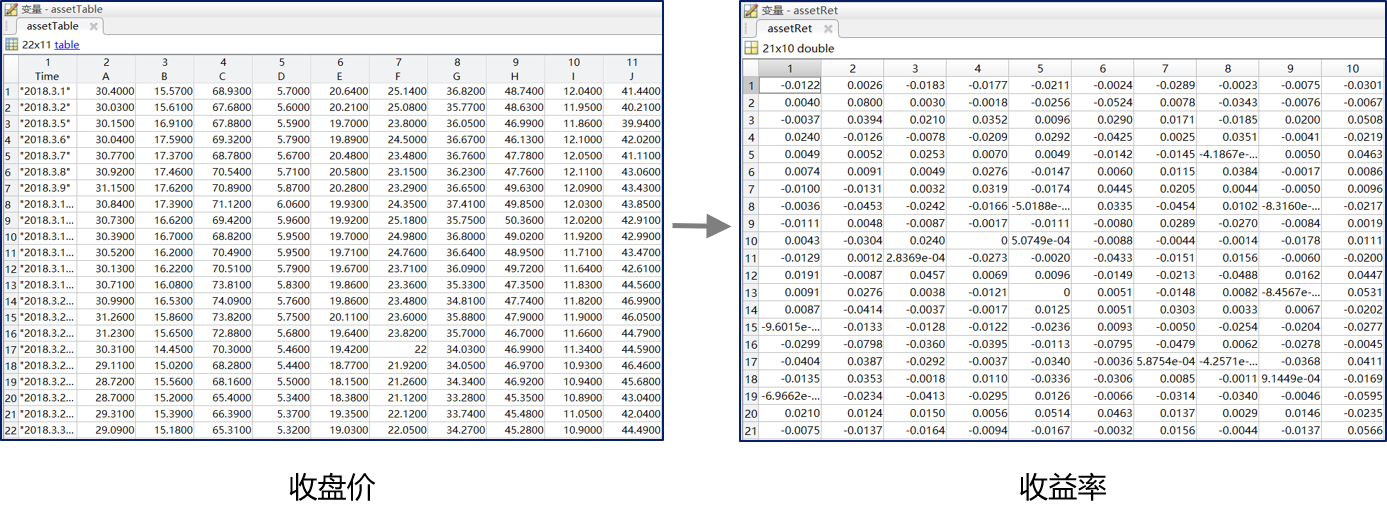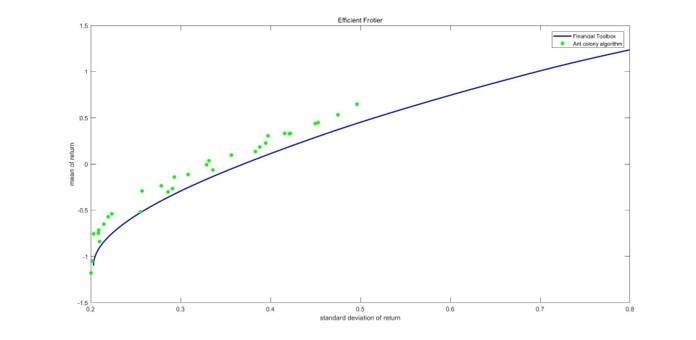【项目】基于蚁群算法的证券投资组合优化

1952年，马科维茨首次提出均值-方差模型(Markowitz Mean-Variance Model)。该模型利用股票的历史交易数据，定义了股票的预期风险与收益，并引入“无差异曲线”和“有效市场边界”的概念，最终导出了最佳投资组合的计算公式。

研究背景1. 一只蚂蚁代表了一个投资组合；
2. 在投资组合优化问题中的蚁群算法要采用连续域优化；
3. 相较于传统蚁群问题，该算法中信息素应该在城市而非路径上积累；
4. 可在蚂蚁移动过程中加入随机扰动以增加全局搜索能力。

研究方法

（一）马科维茨投资组合理论简介

• 单一证券i的预期收益: $E\left(r_{i}\right)=\sum_{s=1}^{n} r_{i s} * P_{s}$

• 证券组合的预期收益: $\overline{r_{p}}=\sum_{i=1}^{N} x_{i} * E\left(r_{i}\right)$

• 单一证券i的预期风险: $\sigma_{\mathrm{i}}=\left(\sum_{s=1}^{n}\left[r_{i s}-E\left(r_{i}\right)\right]^{2} * P_{S}\right)^{\frac{1}{2}}$

• 证券组合的预期风险: $\sigma_{\mathrm{p}}=\left(\sum_{i=1}^{N} \sum_{j=1}^{N} \operatorname{cov}_{i j} * x_{i} * x_{j}\right)^{\frac{1}{2}}$

1. 对每一水平的风险，该组合提供最大的预期收益；
2. 对每一水平的预期收益，该组合能提供最小的风险。（二）蚁群算法在马科维茨模型中的应用研究模型

（一）模型假设

1. 假设投资者选择n只证券，则每个投资组合可视为一个n维单位向量$𝑃=\{𝑥_1,𝑥_2,⋯,𝑥_𝑛\}$，其中$𝑥_𝑖$表示第𝑖只证券所占权重，$x_𝑖>0$
2. 假设在蚁群中有m只蚂蚁，每只蚂蚁都代表一个投资组合P。蚂蚁移动的过程，相当于从一个投资组合变换到另一个投资组合。
3. 目标函数空间是一个连续的r-σ二维空间，其值由每个投资组合P的收益率的均值方差决定。其中r代表收益率均值，σ代表收益率的标准差。
4. 该模型采用的是单期方法，即在t=0时刻买入一个资产组合，在t=1时卖出。
5. 信息素在蚂蚁身上积累，越接近有效边界的蚂蚁，释放的信息素越多。蚂蚁对身边一定范围内的其他蚂蚁有吸引作用，且自身积累的信息素越多，吸引越强。
6. 交易没有最小交易单位限制，即能以分数股的形式交易。
7. 交易中无交易成本。
8. 交易不允许卖空。(即$𝑥_𝑖≥0$)

（二）模型改进：多元函数连续域蚁群算法Note: 众所周知，绘图最高只能画到三维，恕我无法直观地表达n元函数连续域蚁群算法的效果。但是最终效果可以从求解马科维茨均值方差模型的近似求解中看出来。详细效果见后文

（三）模型特点

（四）寻优步骤

1. 初始化蚂蚁。算法会根据信息素，确定每只蚂蚁对应的转移概率。
2. 本文以转移概率作为衡量指标。按照转移概率大小的不同，分别选择局部搜索策略和全局搜索策略。当转移概率较小时，说明当前蚂蚁比较接近最优蚂蚁，采用局部搜索策略；当转移概率较大时，采用全局搜索策略。
3. 计算搜索产生的新投资组合的均值和方差，判断新组合和原组合解的帕累托关系，如果新组合优于原组合，则用新组合的投资组合P替代原组合。
4. 根据出现的新投资组合，计算本轮信息素。考虑挥发系数Rou，将上轮信息素乘以(1-Rou)，在加上本轮新增信息素，得出迭代至下一轮的信息素向量。

研究成果

（一）原始数据（二）计算结果